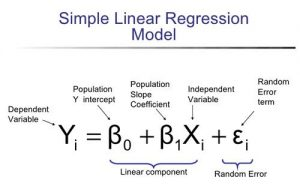What is Linear Regression?

Linear regression is a basic and commonly used type of predictive analysis

FYI, Predictive analytics involves extracting data from existing data sets with the goal of identifying trends and patterns. These trends and patterns are then used to predict future outcomes and trends. Predictive analytics is the branch of the advanced analytics which is used to make predictions about unknown future events. Predictive analytics uses many techniques from data mining, statistics, modeling, machine learning, and artificial intelligence to analyze current data to make predictions about future. It uses a number of data mining, predictive modeling and analytical techniques to bring together the management, information technology, and modeling business process to make predictions about future. The patterns found in historical and transactional data can be used to identify risks and opportunities for future. Predictive analytics models capture relationships among many factors to assess risk with a particular set of conditions to assign a score, or weightage.The overall idea of regression is to examine two things:

(1) does a set of predictor variables do a good job in predicting an outcome (dependent) variable?

(2) Which variables in particular are significant predictors of the outcome variable, and in what way do they–indicated by the magnitude and sign of the beta estimates–impact the outcome variable?

These regression estimates are used to explain the relationship between one dependent variable and one or more independent variables.  The simplest form of the regression equation with one dependent and one independent variable is defined by the formula y = c + b*x, where y = estimated dependent variable score, c = constant, b = regression coefficient, and x = score on the independent variable.

There are several types of linear regression analyses available to researchers.

• Simple linear regression
1 dependent variable (interval or ratio), 1 independent variable (interval or ratio or dichotomous)

• Multiple linear regression
1 dependent variable (interval or ratio) , 2+ independent variables (interval or ratio or dichotomous)

• Logistic regression
1 dependent variable (dichotomous), 2+ independent variable(s) (interval or ratio or dichotomous)

• Ordinal regression
1 dependent variable (ordinal), 1+ independent variable(s) (nominal or dichotomous)

• Multinominal regression
1 dependent variable (nominal), 1+ independent variable(s) (interval or ratio or dichotomous)

• Discriminant analysis
1 dependent variable (nominal), 1+ independent variable(s) (interval or ratio)

When selecting the model for the analysis, an important consideration is model fitting.  Adding independent variables to a linear regression model will always increase the explained variance of the model (typically expressed as R²).  However, overfitting can occur by adding too many variables to the model, which reduces model generalizability.  Occam’s razor describes the problem extremely well – a simple model is usually preferable to a more complex model.  Statistically, if a model includes a large number of variables, some of the variables will be statistically significant due to chance alone.

Article Source: statisticssolutions